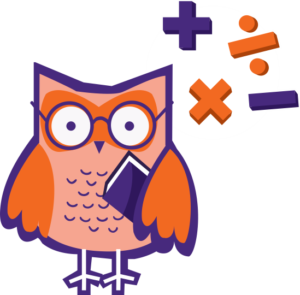Mathematics — britishschool-online.com

Designed and built with care, filled with creative elements

##### Math vocabulary: About 100 words and phrases.
Audio: 1 module. Number and Place Value
m
m
m
m
m
m
m
m
m
m
m
m
m
m
m
m
m
m
m
m
m
m
m
m
m
m
m
m
m
m
m
m
m
m
m
4 Questions
##### Math vocabulary: About 150 words and phrases
1 audio
Audio: 1 module. Number and Place Value
m
Top

### Mathematics

Basic subjects of primary school

## MathematicsCategories:iPrimary Maths programme is based on the latest English National Curriculum (2014) and provides a strong foundation in Mathematics. With its exciting games, practice activities, tricky problems, the course can easily capture children’s interest and inspire a genuine love of mathematics. The elements of mental arithmetic (abacus) are built in the course which helps to develop children’s memory, speed of thinking and lets them remain focused and engaged during a lesson. Content-language integrated approach(CLIL) and immersion into the English language environment helps to master students’ English and easily acquire all the necessary mathematics vocabulary.

### What resources are used at Math lessons?

The course is based on the UK Primary mathematics toolkit called Abacus This unique resource, designed by Pearson, ensures understanding and progression for every student and inspires their genuine love to maths. It includes colourful presentations, interactive tasks, problem-solving activities and maths games.

###### What level of English do students need to study Math in English?

To start the course of iPrimary Mathematics (Year1 and Year2) students need to have A1 (Beginner) level of English: understand and use some basic phrases, ask and answer simple questions, read and write numbers. This will be enough to start the course. And only after the basic numbers are revised, the students will be introduced to new terms and operational words, the names of shapes and basic measures. To join the course in Year3 and Year4, the students need to have Elementary level of English (A1-A2), and the students of Year 5 and Year 6 are supposed to have at least Pre-Intermediate level of English. They need to use English to read graphs, understand word problems and comment on their actions.

#### Year 1-2

 4 main modules: “Number and Place Value”, “Addition and Subtraction”, “Geometry”, “Measures”. Math vocabulary: About 100 words and phrases. • count to 100; read and write numbers from 1 to 20, • add, subtractcompare numbers within 100; • distinguish between odd and even numbers; •solve one-stage problems of addition and subtraction, represent graphically the conditions; •know the names of 2D shapes and their main properties; • know and find the main types of symmetry; • measure length or height; • sort objects on the Venn diagram.

#### Year 3-4

 5 main modules: “Addition and Subtraction”,  “Multiplication and Division”, “Geometry”, “Simple and Decimal Fractions, Coefficients, Percentages”,  “Measurements”. Math vocabulary: About 150 words and phrases. • add and subtract 2-digit numbers to 100, round up to tens and hundreds, break the numbers into digits; •multiply and divide 1-digit and 2-digit numbers • take measurements and convert some units of time, length, weight; • know the names of the months, days of the week, the number of days in each month, year and leap year;  tell and record time, • know, recognize and create various 3D-forms, know their main properties; • find the parts of an integer; • solve complex problems on addition, subtraction, multiplication and division; find time, cost, distance, weight.

#### Year 5-6

 7 main modules: “Properties of numbers”,  “Addition and subtraction”,  “Multiplication and division”,  “Geometric figures”, “Simple and decimal fractions, percentages, proportions”,  “Measurements”, “Statistics”. Math vocabulary: About 200 words and phrases. • read, write, compare, round numbers to 1,000,000; • add and subtract integers with more than four digits; • multiply and divide numbers using mental and graphical methods; • add, subtract, multiply, divide and compare fractions; • convert units of measurement; • know various 3-d shapes and their properties; • find the area and perimeter of ​​ rectangles; • measure, draw and compare angles; • draw reflected shapes; • read graphs and tables; • solve complex multistage problems.

#### Price

 iPrimary (5-7 y.o.) Year 1, 2 iPrimary (7-9 y.o.)  Year 3, 4 iPrimary (9-11y.o.) Year 5, 6 3 subjects: Mathematics, Science and English 3 subjects: Mathematics, Science and English 3 subjects: Mathematics, Science and English 36 lessons on each subject, every lesson lasts 30 minutes 36 lessons on each subject, every lesson lasts 45 minutes 36 lessons on each subject, every lesson lasts 50 minutes Price of the course (3 subjects) 1155 EUR Price of the course (3 subjects) 1710 EUR Price of the course (3 subjects) 1920 EUR Registration fee 100 EUR Registration fee 150 EUR Registration fee 200 EUR

At the end of iPrimary Programme (Year 6) the students can take exams in Math, Science and English and get a world-renowned iPrimary certificates.

### Course Curriculum

Develops children’s memory and their speed of thinking

##### Math vocabulary: About 100 words and phrases.

• count to 100; read and write numbers from 1 to 20, • add, subtractcompare numbers within 100; • distinguish between odd and even numbers; •solve one-stage problems of addition and subtraction, represent graphically the conditions; •know the names of 2D shapes and their main properties; • know and find the main types of symmetry; • measure length or height; • sort objects on the Venn diagram.

Audio: 1 module. Number and Place Value
m
m
m
m
m
m
m
m
m
m
m
m
m
m
m
m
m
m
m
m
m
m
m
m
m
m
m
m
m
m
m
m
m
m